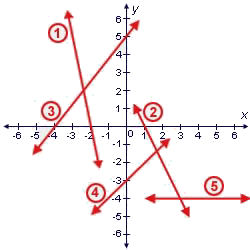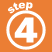Home    |    Teacher    |    Parents    |    Glossary    |    About UsType the number of the line that matches the slope or y-intercept. slope = -5y-intercept = -3 slope = 0 y-intercept = 5 slope = -2
 Homework Help | Algebra | Graphing Equations and InequalitiesEmail this page to a friend·  The coordinate plane·  Slope and y-intercept·  Graphing linear     equationsFirst Glance In Depth Examples WorkoutSlope and y-intercept